Olympiad Test Level 1: Numbers’ Magic- 2

# Olympiad Test Level 1: Numbers’ Magic- 2 - Class 3

Test Description

## 15 Questions MCQ Test GK Olympiad for Class 3 - Olympiad Test Level 1: Numbers’ Magic- 2

Olympiad Test Level 1: Numbers’ Magic- 2 for Class 3 2023 is part of GK Olympiad for Class 3 preparation. The Olympiad Test Level 1: Numbers’ Magic- 2 questions and answers have been prepared according to the Class 3 exam syllabus.The Olympiad Test Level 1: Numbers’ Magic- 2 MCQs are made for Class 3 2023 Exam. Find important definitions, questions, notes, meanings, examples, exercises, MCQs and online tests for Olympiad Test Level 1: Numbers’ Magic- 2 below.
 1 Crore+ students have signed up on EduRev. Have you?
Olympiad Test Level 1: Numbers’ Magic- 2 - Question 1

### Study the given patterns carefully and find the number of ice-cream cones in Pattern 4.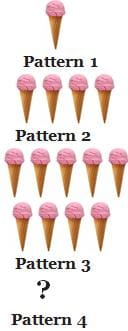Detailed Solution for Olympiad Test Level 1: Numbers’ Magic- 2 - Question 1

Each pattern has number of ice-creams equal to the product of the pattern number by itself.
1 × 1 = 1
2 × 2 = 4
3 × 3 = 9
So, 4 × 4 = 16

Olympiad Test Level 1: Numbers’ Magic- 2 - Question 2

### Arrange the following words in the question in a logical sequence. (1) Evening (2) Night (3) Morning (4) Afternoon

Detailed Solution for Olympiad Test Level 1: Numbers’ Magic- 2 - Question 2

The day starts with morning, then moves on to afternoon, evening and ends with night.

Olympiad Test Level 1: Numbers’ Magic- 2 - Question 3

### Find the missing number in the given number pattern below.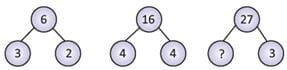Detailed Solution for Olympiad Test Level 1: Numbers’ Magic- 2 - Question 3

The number in the upper circle is the product of the numbers in the lower two circles.
3 × 2 = 6
4 × 4 = 16
So, 9 × 3 = 27

Olympiad Test Level 1: Numbers’ Magic- 2 - Question 4

A big water bottle can hold 2 liters of water. It is being filled by the mugs of different capacities. Which of the following combinations of mugs can fill the bucket?

Detailed Solution for Olympiad Test Level 1: Numbers’ Magic- 2 - Question 4

(250 mL + 250 mL + 250 mL + 250 mL) + (500 mL + 500 mL) = 1 L + 1 L = 2 L

Olympiad Test Level 1: Numbers’ Magic- 2 - Question 5

What number does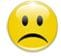stand for?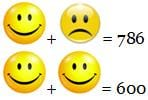Detailed Solution for Olympiad Test Level 1: Numbers’ Magic- 2 - Question 5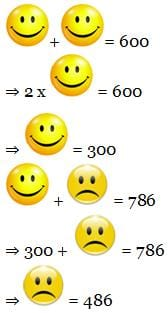Olympiad Test Level 1: Numbers’ Magic- 2 - Question 6

Find the sum of the sides of a badminton court which is 13 meters long and 6 meters wide?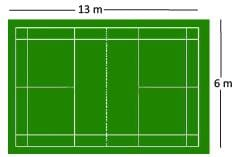Detailed Solution for Olympiad Test Level 1: Numbers’ Magic- 2 - Question 6

Sum of sides = 13 + 6 + 13  + 6 = 38 meters

Olympiad Test Level 1: Numbers’ Magic- 2 - Question 7

Smita has bought a ticket with a number written on it. Identify the number written on Smita’s ticket if the number is 120 more than the smallest 3-digit number?Detailed Solution for Olympiad Test Level 1: Numbers’ Magic- 2 - Question 7

Smallest 3-digit number = 100
Ticket number = 120 + 100 = 220

Olympiad Test Level 1: Numbers’ Magic- 2 - Question 8

Year 2020 was a leap year. How many total days are there in a leap year?

Detailed Solution for Olympiad Test Level 1: Numbers’ Magic- 2 - Question 8

There are 366 days in a leap year.

Olympiad Test Level 1: Numbers’ Magic- 2 - Question 9

What will you get, first add 4125 and 3985, then subtract 0 from it and then multiply it by 0?

Detailed Solution for Olympiad Test Level 1: Numbers’ Magic- 2 - Question 9

Anything multiplied by 0 gives the product as 0.

Olympiad Test Level 1: Numbers’ Magic- 2 - Question 10

Which of these is NOT a correct example of 3-dimensional shape?

Detailed Solution for Olympiad Test Level 1: Numbers’ Magic- 2 - Question 10

Coins are circular in shape which means they are twodimensional.

Olympiad Test Level 1: Numbers’ Magic- 2 - Question 11

Which unit can be used to measure a glass of juice?

Detailed Solution for Olympiad Test Level 1: Numbers’ Magic- 2 - Question 11

Milliliters are used to measure small amounts of liquids.
1 liter = 1000 milliliter

Olympiad Test Level 1: Numbers’ Magic- 2 - Question 12

How many times can you take away 6 from 72?

Detailed Solution for Olympiad Test Level 1: Numbers’ Magic- 2 - Question 12

72 ÷ 6 = 12

Olympiad Test Level 1: Numbers’ Magic- 2 - Question 13

Which day will be the 35th day from 20 September 20XX?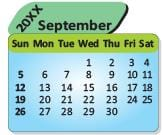Detailed Solution for Olympiad Test Level 1: Numbers’ Magic- 2 - Question 13

Starting from Monday, 7th, 14th, 21st, 28th and 35th day fall on Sunday.

Olympiad Test Level 1: Numbers’ Magic- 2 - Question 14

The number which divides in a division is called the _________.

Detailed Solution for Olympiad Test Level 1: Numbers’ Magic- 2 - Question 14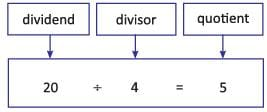Olympiad Test Level 1: Numbers’ Magic- 2 - Question 15

Look at this picture of a flower. It has been divided into equal parts. What fraction do shaded parts represent?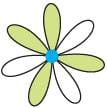Detailed Solution for Olympiad Test Level 1: Numbers’ Magic- 2 - Question 15

= 4
Total parts = 7
Fraction = 4/7

48 tests